# Nearly spanning regular subgraphs

 Importance: High ✭✭✭
 Author(s): Alon, Noga Mubayi, Dhruv
 Subject: Graph Theory » Basic Graph Theory
 Keywords: regular subgraph
 Posted by: mdevos on: May 22nd, 2008
Conjecture   For everyand every positive integer, there existsso that every simple-regular graphwithhas a-regular subgraphwith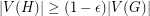.

Petersen's theorem asserts that every regular graph of even degree contains a 2-factor (i.e. a spanning 2-regular subgraph). Iterating this easy result we find that for any pair of positive even integers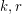, every-regular graph has a spanning-regular subgraph. The cases when eitheroris odd are considerably more complicated. There are some nice general results (see [AFK]) which show that every regular graph of sufficiently high degree contains a-regular subgraph. However these theorems give no bound on the size of this subgraph.

For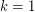this conjecture is an easy consequence of Vizing's Theorem. Indeed, this theorem implies that every-regular graphhas a 1-regular subgraphwith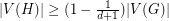(just choose a largest color class from a-edge coloring). Alon [A] proved the conjecture for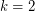with the help of two famous results on permanents: the Minc Conjecture (proved by Bregman), and the van der Waerden conjecture (proved by Falikman and Egorichev). It is open for all.

## Bibliography

*[A] N. Alon, Problems and results in extremal combinatorics, J, Discrete Math. 273 (2003), 31-53.

[AFK] N. Alon, S. Friedland and G. Kalai, Regular subgraphs of almost regular graphs, J. Combinatorial Theory, Ser. B 37(1984), 79-91.

* indicates original appearance(s) of problem.